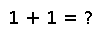digamma

digamma(x)

Compute the digamma function of x (the logarithmic derivative of gamma(x))

Examples

Checking you are not a robot: